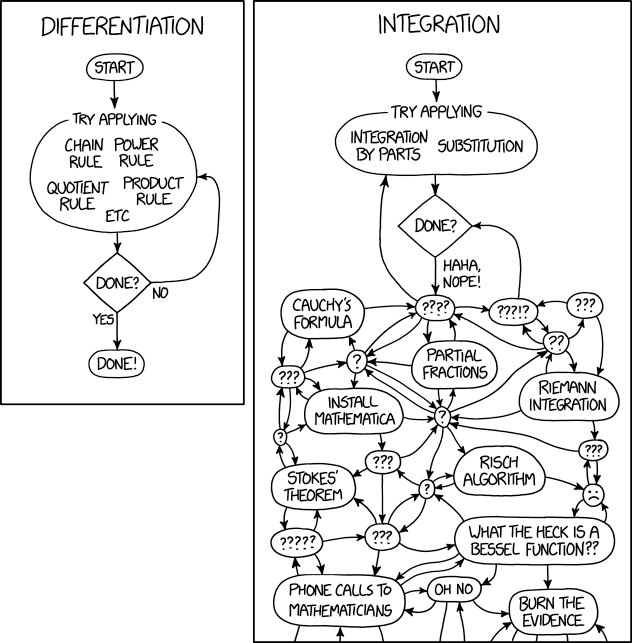# To Save The Science Poster, Researchers Want To Kill It And Start Over

Professional conferences often feature poster sessions, and, more often than not, the poster is simply incomprehensible to somebody walking through the aisles.So I enjoyed this article about an innovative way to bring scientific posters in the 21st century. The money quote:

“The current method is not effective in communicating research findings. For instance, in my field, we all want improvements in our life: vaccines for all diseases, easier delivery of vaccines, innovative way to finance vaccines, effective ways tackling vaccine hesitancy,” Suharlim says. “Experts are all coming to these conferences, and they have limited time to update their knowledge.”

The proof is definitely in the pudding:

Finally, here’s a YouTube video explaining the concept:

# Sum of Three Cubes

I now have a new example of an existence proof to show my students.

Last year, mathematicians Andrew Booker and Andrew Sutherland found solutions to the following two equations:$x^3 + y^3 + z^3 = 33$ and$x^3 + y^3 + z^3 = 42$. The first was found by Booker alone; the latter was found by the collaboration of both mathematicians. These deceptively simple-looking equations were cracked with a lot of math and a lot of computational firepower. The solutions:$(8,866,128,975,287,528)³ + (–8,778,405,442,862,239)³ + (–2,736,111,468,807,040)³ = 33$

$latex (–80,538,738,812,075,974)3 + 80,435,758,145,817,5153 + 12,602,123,297,335,6313 = 42$

At the time of this writing, that settles the existence of solutions of$x^3 + y^3 + z^3 = n$ for all positive integers$n$ less than 100. For now, the smallest value of$n$ for which the existence of a solution is not known is$n = 114$.

For further reference, including links to the original articles by Booker and then Booker and Sutherland, please see:

# Differentiation and Integration

As I tell my calculus students, differentiation is a science. There are rules to follow, but if you follow them carefully, you can compute the derivative of anything. This leads to one of my favorite classroom activities. However, integration is as much art as science; for example, see my series on different techniques for computing$\displaystyle \int_0^{2\pi} \frac{dx}{\cos^2 x + 2 a \sin x \cos x + (a^2 + b^2) \sin^2 x}$

The contrast between differentiation and integration was more vividly illustrated in a recent xkcd webcomic:Source: https://xkcd.com/2117/

# A Professor Asked His Students to Write Their Own Exam Questions

I was intrigued by this article in the Chronicle of Higher Education about professors who asked students to write their own exam questions, thus forming a test bank from which the actual exam would be constructed. I’m not sure if I’d try this myself, but it definitely gave me food for thought.

# What Industrial Jobs Can I Get With a Math Degree?

From Thomas Network:

While not every math major will get the chance to work on something as exciting as the Enigma Code or black holes, there’s one career that will provide an endless stream of fascinating challenges to keep even the brightest mathematical minds busy: manufacturing.

Mathematicians are in demand for these four skills in particular:

1. Analytical skills
2. Problem-solving skills
3. Critical-thinking skills
4. Quantitative reasoning skills

The U.S. Bureau of Labor Statistics expects the demand for math majors to grow by 30% from 2018 to 2028. As of last year, the median annual wage for mathematicians was \$101,900.

# Visualizing Vectors

From the Math Values blog of the Mathematical Association of America:

Anyone who has taught linear algebra knows how easy it is for students to get absorbed in performing matrix computations and memorizing theorems, losing the beauty of the structures in this foundational subject. James Factor and Susan Pustejovsky of Alverno College in Milwaukee, WI, bring back the visual beauty of linear algebra through their NSF-funded project Transforming Linear Algebra Education with GeoGebra Applets.

The applets are freely available in the GeoGebra book Transforming Linear Algebra Education https://www.geogebra.org/m/XnfUWvvp. Each topic is packaged with a video to show how the applets work, the applet, and learning activities.